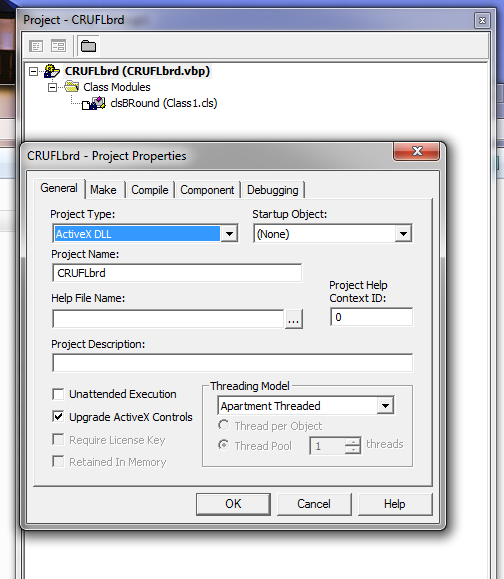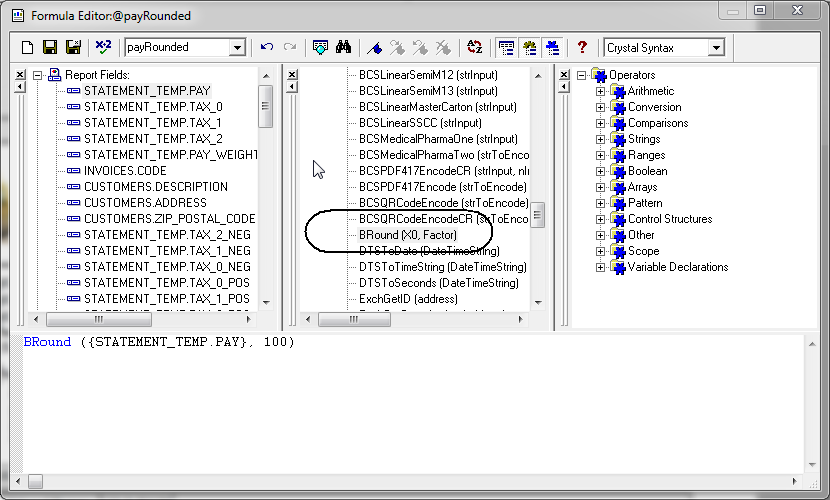# The Salty Economist

Things I Should Have Learned in High School

## Adding Bankers Rounding Function to Crystal Reports

We use bankers' rounding in much of our software.

For those of you who don't know what banker rounding is, it is commonly known as round to even.  For example if you have a number like 105.5550 and wand to round it to 2 decimal places, bankers' rounding would give you 105.56 because 6 is even and 5 is odd.  Likewise, if you had the number 105.5450, bankers' rounding would yield 105.54 because 4 is even.  The reason to use bankers' rounding is that it is more "fair" than always rounding .5 up or down.  Bankers' rounding is intended to round up half the time and round down half the time.  This type of rule seems to be the most neutral way to round numbers.

I find it interesting that all software languages have rounding functions, but their documentation rarely tells you their rounding rules.  Most of the time you just have to figure it out yourself.  Also, because real numbers are usually not stored with exact precision, rounding of numbers ending 0.5 is fraught with problems.

For example, the real number 105.555 is sometimes stored as 105.55499999999, which would always be rounded down.

In Crystal Reports (8.5) there is a rounding function 'Round' that appears to always round up.  Because of this, I wanted to see if I could add a function to implement bankers rounding in Crystal Reports.

After some sleuthing about, I found a really cool way to do this.

It turns out that since version 6 of Crystal Reports, you the ability of creating a user-defined formula using any language that can create a COM application. Voila! VB6.  This means I can basically do anything I want in Crystal Reports.  This is so Cool.

Let's take a quick look at how your COM application interacts with Crystal Reports.  One of the DLL's installed by Crystal Reports is a file called U2LCOM.DLL.  This DLL acts as a gateway between your COM application and Crystal Reports.  U2LCOM.DLL looks for any COM applications that follow Crystal's specific naming convention for user defined functions.  It then displays any public functions from your COM application in the "Additional Functions" category in the Crystal formula editor window.  That is really all there is to it.

Here are the rules you must follow.

(1) The COM application's file name must follow a strict naming convention. It must be exactly 8 characters in length and prefixed with "CRUFL".  This means you get the last 3 digits to make up your name.

(2) The COM application must not contain any public functions by the same name as existing Crystal Report functions.

(3) The U2LCOM.DLL from Version 8.5 has a limit of 300 functions.  This is the total number of functions from all UFLs, not just yours alone.

Bankers' Rounding Example

(1)  In VB6, create a new activeX dll project.As shown above, I have a VB6 project named CRUFLbrd - "CRUFL" + 3 digits "brd"

I have one class:  clsBRound.  There do not appear to be any limitations of the naming convention for a class.

Here is my Class Code:

------------------------------------------------------------------------------------------------------------

Option Explicit

Public UFPrefixFunctions As Boolean

Private Sub Class_Initialize()

UFPrefixFunctions = False

End Sub

Public Function BRound(ByVal X0 As Double, ByVal Factor As Double) As Double

'  For smaller numbers:
'  BRound = CLng(X * Factor) / Factor

Dim Temp As Double
Dim FixTemp As Double
Dim n As Long
Dim zeta As Double
Dim X As Double

On Error GoTo Err_Handler

X = Abs(X0)

If Abs(X) > 1# Then
n = Fix(Log10(Abs(X)))
zeta = 0.00000000001 * Pow10(10, n)
Else
zeta = 0.00000000001
End If

Temp = (X * Factor)
FixTemp = Fix(Temp + 0.5 * Sgn(X) + zeta)

' Handle rounding of .5 in a special manner

If Abs(Temp - Int(Temp) - 0.5) < zeta Then
If FixTemp / 2 <> Int(FixTemp / 2) Then ' Is Temp odd
' Reduce Magnitude by 1 to make even
FixTemp = FixTemp - Sgn(X)
End If
End If

BRound = (FixTemp / Factor) * Sgn(X0)

Exit Function

Err_Handler:

End Function

Private Function Log10(X) As Double

Log10 = Log(X) / Log(10#)

End Function

Private Function Pow10(aVal As Double, dec As Long)

Pow10 = Exp(dec * Log(aVal))

Exit Function

Err_Handler:

End Function

------------------------------------------------------------------------------------------------------------

The first part of the class code:

Public UFPrefixFunctions As Boolean

Private Sub Class_Initialize()

UFPrefixFunctions = False

End Sub

Performs an important role.  The UFPrefixFunctions property is used by Crystal to use a default naming convention.  By default this property is True and will name your function using the class name, the letters "UFL" and your function name.  In my example, the default naming convention would generate a name: "clsBRoundUFLBround" -- now there's a mouthful.  This is the name that would appear in under the additonal functions heading in the formula window is Crystal.  I don't like this name.  I just want it to be named "BRound," thank you very much.  By setting UFPrefixFunctions = False, I can ensure that Crystal does NOT append the awkward prefix and just uses the name BRound.  Excellent.

I compile my project and I am ready to go.  Note, the compile process in VB6 will also register your COM object, so you don't need to register it separately.  But if you want to distribute it, you will need to register it before you can use it.  Obviously, if you are using a function from the .dll in an actual report, you will have to install the new .dll along with the other Crystal runtime components.

So Now when I open Crystal and go the formulas window, I see my function!!!Somehow, Crystal searches the registry for classes with their precise naming convention and loads them up when you open Crystal.  You don't have to manually add anything...it is all automatic.

Sweet.  That's all there is to it.

Now on with the tedium of the Bankers Rounding code.

First, a couple of requirements:

(1)  The function must handle any number of rounded decimal places.

(2)  It must handle Negative numbers.

The function itself is called with 2 parameters:  The number you want to round and a "Factor" for how many decimal places you want to round to.  In my function, you would pass in the number 10 for one decimal place, 100 would be for two decimal places, 1000 would be three places and so on.

Let's work through the code with an example:  104.655 rounding to 2 decimal places.

This part:

X = Abs(X0)

If Abs(X) > 1# Then
n = Fix(Log10(Abs(X)))
zeta = 0.00000000001 * Pow10(10, n)
Else
zeta = 0.00000000001
End If

begins by using the absolute value of the number passed in -- 104.655.

For a number greater than 1 (which ours is), I then want to create a value 'zeta' that is close to zero, but not zero.  The use for this is for handling imprecise real numbers like 104.654999999..., that I really want to treat as 104.655.

The line n = Fix(Log10(Abs(X))) takes the log (base 10) of 104.655 which is something like 2.02, and then returns only the integer part.

So, n = 2.  We then multiply 0.00000000001 by 10 raised to the power of 2 (=100), yielding a zeta of 0.000000001
The use for zeta will be clear down below.

Next we create 2 temp variables Temp and FixTemp:

Temp = (X * Factor)
FixTemp = Fix(Temp + 0.5 + zeta)

Temp takes our number 104.655 and multiplies it by 100, yielding 10465.5

FixTemp takes Temp and adds 0.5 (yielding 10466.0) and adds zeta, yielding 10466.000000001.  We then grab the integer part or 10466 (exactly)

Note (1): if we were rounding -104.655, Temp would still be 10465.5 and FixTemp would be the integer part of 10466.000000001 or 10466.0. Recall, from the beginning that we are dealing with all positive numbers.  We flip the sign at the end.

Note (2): if we were rounding 104.654999999...,  Temp would be 10465.4999999... and FixTemp would be something like 10466.000000000999. Again, we then grab the integer part or 10466.  The purpose of zeta is to prevent the returning of a FixTemp value of 10465.

Now, we have 2 cases:

(1)  The mantissa of Temp is .5

(2)  Or it is not.

Case 2:  Simple.  Just return FixTemp divided by Factor (100) and multiplied by the sign of X0.

So, if our number had been 104.664, Temp would be 10466.4 and FixTemp would be 10466.0.   We would return 104.66 ( = (10466.0 / 100.0) * 1)

Case 1:  Harder.

First, calculate the mantissa of Temp.

= Temp - Int(Temp).  Then take its absolute value and compare it to 0.5.

We do this comparison be taking the difference between Temp - Int(Temp) and 0.5 and then checking to see if the difference is arbitrarily small (meaning less than zeta).

Thus the expression Abs(Temp - Int(Temp) - 0.5) < zeta.

In our example this is Abs(10466.5 - 10466 - 0.5) < .000000001.  This is TRUE.

With the number -104.665, we would have the same result (recall...all positive numbers).

We use the zeta factor to stay away from the imprecise equality comparisons between two real numbers.

In the case of 104.654999999...,  Temp would be something like 10465.4999999...

And the expression Abs(10465.4999999... - 10466 - 0.5) < .000000001. Would also be TRUE.

OK, so now we know the mantissa is 0.5. How do we handle, the odd/even of bankers rounding?

Look at this expression:

If FixTemp / 2 <> Int(FixTemp / 2) Then ' Is Temp odd
' Reduce Magnitude by 1 to make even
FixTemp = FixTemp - 1
End If

We take FixTemp and divide it by 2.  In our example, FixTemp is 10466.0.  Dividing by 2, we get 5233.

The Int(5233) equals 5233, which is equal to 5233.

So we do nothing and then return 104.66 = 10466.0 / 100. = FixTemp / 100.

Thus, we round 104.655 up to 104.66

If our number had been 104.665 (instead of 104.655), then FixTemp is 10467.0 and dividing by 2 yields 5233.5.  This does not equal Int(5233.5), so we deduct 1 from FixTemp and return 104.66 = 10466.0 / 100. = FixTemp / 100

Thus, we round 104.665 down to 104.66

For negative -104.655 (instead of 104.655), FixTemp is still 10466.0 and dividing by 2 yields 5233.

So we do nothing and then return -104.66 = (10466.0 / 100.) * Sgn(X0).  The Sgn(X0) = -1.

Thus, we round -104.655 down to -104.66

Likewise, for negative -104.665, we return -104.66.

The moral, all .5's round to the nearest even number.

Print | posted on Saturday, June 28, 2014 11:04 AM |